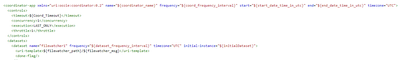Support Questions

# Oozie Dataset Frequency is more than the Coordinator Frequencyprava_edu
New Contributor

I have a coordinator with Datasets. I would like to know how exactly the Dataset frequency will work.

I have tried triggering the coordinator by setting below frequencies and didn't see any difference.

Example 1: Cood_frequency_interval = 60 (mins) and dataset_frequency_interval = 30 (mins)

Example 2: Cood_frequency_interval = 60 (mins) and dataset_frequency_interval = 120 (mins).